International
Tables for
Crystallography
Volume A
Space-group symmetry
Edited by M. I. Aroyo

International Tables for Crystallography (2015). Vol. A, ch. 1.5, pp. 88-90

## Section 1.5.3.2.1. Transformations between different settings of P21/c

G. Chapuis,d H. Wondratscheka and M. I. Aroyob

#### 1.5.3.2.1. Transformations between different settings of P21/c

| top | pdf |

In the space-group tables of this volume, the monoclinic space group(14) is described in six different settings: for each of the unique axis b' and unique axis c' settings there are three descriptions specified by different cell choices (cf. Section 2.1.3.15). The different settings are identified by the appropriate full Hermann–Mauguin symbols. The basis transformations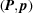between the different settings are completely specified by the linear part of the transformation, the 3 × 3 matrix P [cf. equation (1.5.1.4)], as all settings ofrefer to the same origin, i.e.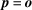. The transformation matrices P necessary for switching between the different descriptions ofcan either be read off directly or constructed from the transformation-matrix data listed in Table 1.5.1.1.

(A) Transformation from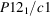(unique axis b, cell choice 1) to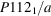(unique axis c, cell choice 1). The change of the direction of the screw axisindicates that the unique direction b transforms to the unique direction c, while the glide vector along c transforms to a glide vector along a. These changes are reflected in the transformation matrix P between the basis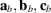ofand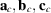of, which can be read directly from Table 1.5.1.1: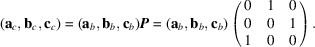• (i) Transformation of point coordinates. From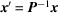, cf. equation (1.5.1.5), it follows that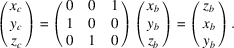For example, the representative coordinate triplets of the special Wyckoff position 2doftransform exactly to the representative coordinate triplets of the special Wyckoff position 2dof: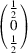and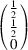transform toand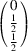.

• (ii) Transformation of the indices in the Reflection conditions' block. Under a coordinate transformation specified by a matrix P, the indices of the reflection conditions (Miller indices) transform according to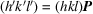, cf. equation (1.5.2.2). The transformation under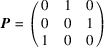of the set of general or special reflection conditions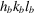forshould result in the set of general or special reflection conditions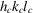of: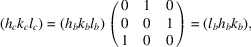i.e.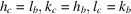(see Table 1.5.3.1).

 Table 1.5.3.1| top | pdf | Transformation of reflection-condition data for P121/c1 to P1121/a
P121/c1P1121/aGeneral conditions h0l: l = 2n hk0: h = 2n
0k0: k = 2n 00l: l = 2n
00l: l = 2n h00: h = 2n

Special conditions for the inversion centres hkl: k + l = 2n hkl: h + l = 2n
• (iii) Transformation of the matrix–column pairsof the symmetry operations. The matrices of the representatives of the symmetry operations ofcan be constructed from the coordinate triplets listed in the general-position block of the group: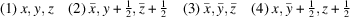Their transformation is more conveniently performed using the augmented-matrix formalism. According to equation (1.5.2.17), the matrices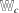of the symmetry operations ofare related to the matrices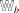ofby the equation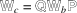, where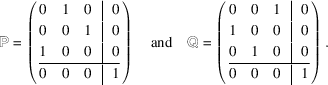The unit matrix representing the identity operation (1) is invariant under any basis transformation, i.e.transforms to. Similarly, the matrix of inversion(3) (the linear part of which is a multiple of the unit matrix) is also invariant under any basis transformation, i.e.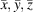transforms to. The symmetry operation (2)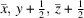, represented by the matrix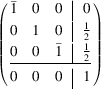transforms to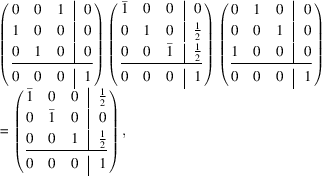which corresponds to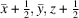.

Finally, the symmetry operation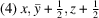represented by the matrix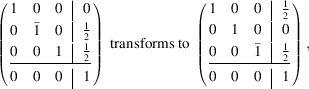corresponding to the coordinate triplet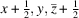[the matrices of (4) and its transformed are those of (2) and its transformed, multiplied by].

The coordinate triplets of the transformed symmetry operations correspond to the entries of the general-position block of(cf. the space-group tables ofin Chapter 2.3).

(B) Transformation from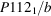(unique axis c, cell choice 3) to(unique axis b, cell choice 1):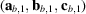=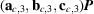. A transformation matrix fromdirectly tois not found in Table 1.5.1.1, but it can be constructed in two steps from transformation matrices that are listed there. For example:

Step 1. Unique axis c fixed: transformation from cell choice 3' to `cell choice 1':Step 2. Cell choice 1 invariant: transformation from unique axis c to unique axis b: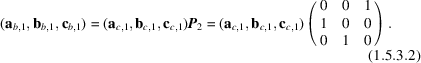The transformation matrix P for the change fromtois obtained by starting from equation (1.5.3.2)and replacing the expression for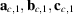with that from equation (1.5.3.1):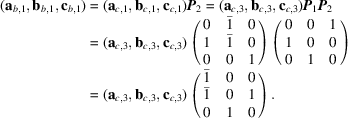The inverse matrix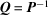can be obtained either by inversion or by the product of the factors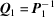and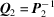but in reverse order: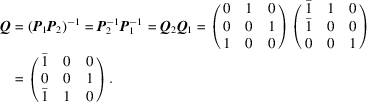The transformation matrix P determined above and its inverse Q permit the transformation of crystallographic data for the change fromto.